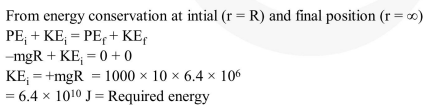# The mass of a spaceship

Question:

The mass of a spaceship is $1000 \mathrm{~kg}$. It is to be launched from the earth's surface out into free space. The value of ' $g$ ' and ' $\mathrm{R}$ ' (radius of earth) are $10 \mathrm{~m} / \mathrm{s}^{2}$ and $6400 \mathrm{~km}$ respectively. The required energy for this work will be :-

1. $6.4 \times 10^{10}$ Joules

2. $6.4 \times 10^{11}$ Joules

3. $6.4 \times 10^{8}$ Joules

4. $6.4 \times 10^{9}$ Joules

Correct Option: 1

Solution: